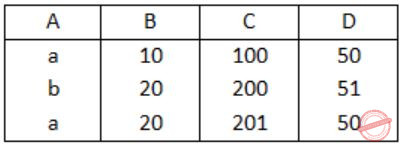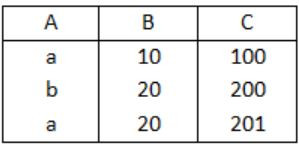October 11, 2023
October 12, 2023
October 11, 2023
###### Software-Engineering
October 12, 2023
 Question 102

Let r be a relation instance with schema R = (A, B, C, D). We define r1 = ΠA,B,C(R) and r2 = ΠA,D(R). Let s = r1*r2 where * denotes natural join. Given that the decomposition of r into r1 and r2 is lossy, which one of the following is TRUE?

 A s ⊂ r B r ∪ s = r C r ⊂ s D r * s = s
Question 102 Explanation:
Let us consider an example:
Table r: R(A, B, C, D)Table r1: ΠA,B,C(R)Table r2: ΠA,D(R)S = r1 * r2 (* denotes natural join)
Table S:Table r ⊂ Table S
⇒ r ⊂ S
Question 102 Explanation:
Let us consider an example:
Table r: R(A, B, C, D)Table r1: ΠA,B,C(R)Table r2: ΠA,D(R)S = r1 * r2 (* denotes natural join)
Table S:Table r ⊂ Table S
⇒ r ⊂ S